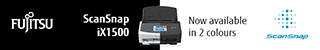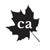Technology Software

# Microsoft Excel wizard and formula tips## Three tricks to increase your efficiency from Alan Salmon of K2E Canada

Author: Alan Salmon

The following three tips from Alan Salmon of K2E Canada uses the Microsoft Excel wizard and formulas to save you time when using spreadsheets. For more tips and tricks from Alan, go the related articles section at the bottom of this page.

### 1. Using Text to Columns to convert text to dates.

Working with dates requires those dates to be in an Excel Date format. Here is how to convert a text date to one of the many Excel Date formats:

•  Select the range of text dates.
•  Click the Data tab.
•  Select Data Tools | Text to Columns and a wizard dialog box will open.
•  In the wizard dialog box, click Next twice to go to Step 3 of the Wizard.
•  Use the default settings in the first two steps of the wizard.
•  In Step 3 of the wizard, select the Date option and select your desired format.
•  Click Finish and the text dates will convert to dates that can be formatted and used in calculations.### 2. Automatically numbering rows.

There are times when you want to insert a row number into column A in your worksheet. The column should reflect the correct number of the row, even when you add or delete rows. There are a number of formulas you can use in column A that will return a row number.

The easiest to use is the ROW function: =ROW(). This formula returns the row number of the cell in which the formula appears. If you want to account for headers in rows 1 and 2 and you want cell A3 to return a row value of 1, then you can modify the formula to reflect the desired adjustment: =ROW()-2.

Another option is to use a formula that actually examines the contents of the adjacent column (B) and return a row number only if there is something in that adjacent cell:

=IF(TRIM(B1)<>"",COUNTA(\$B\$1:B1)&".","")

This formula in cell A1 examines the contents of cell B1. If there is something there, then the COUNTA function is used to count the number of occupied cells between cell B1 and whatever cell is to the right of where this formula is placed. The formula also places a period after the row number that is returned.

Be sure the dollar signs are included, as shown, and then copy the formula down, as many cells as necessary to create your row numbers.

The advantage of a formula such as this one is that it checks to see if something is in column B before it returns a row number. This means that you can copy the formula down beyond the actual end of your data rows, and only those rows that have data (triggered by something in column B) will have a row number. The same sort of technique could be used with the ROW function instead of the COUNTA function.

### 3. Counting the number of blank cells in a range.

If you need to count the number of blank cells in a range here is a little-known formula that will do the trick. One of the worksheet functions provided by Excel allows you to quickly and easily count the number of blank cells in a range. The format of the function is as follows: =COUNTBLANK (range)

The function returns an integer value representing the number of blank cells in the range. Be careful though. If you have the display of zero values suppressed for the worksheet, a cell can appear blank when it is not really blank. COUNTBLANK returns blank cells, not counting those that would have a zero displayed if you chose to display such values.

Alan Salmon is recognized as Canada’s leading analyst in accounting technology. As the founder of K2E Canada Inc., Alan has over 34 years of business, management systems, education and journalism experience. Visit Salmon.ca to learn more.